﻿ Near-infrared Spectrum Detection of Fish Oil DHA Content Based on Empirical Mode Decomposition and Independent Component Analysis

### Near-infrared Spectrum Detection of Fish Oil DHA Content Based on Empirical Mode Decomposition and I...

CAI Jian­huaOPEN ACCESSPEER-REVIEWED

## Near-infrared Spectrum Detection of Fish Oil DHA Content Based on Empirical Mode Decomposition and Independent Component Analysis

Department of Physics and Electronics, Hunan University of Arts and Science, Changde, Hunan, China

### Abstract

The near infrared (NIR) spectrum of fish oil is often very weak, and part of the peaks are submerged in the noise and are difficult to distinguish especially when NIR spectrum is applied to component analysis. A new method is proposed to get the pretreatment of NIR spectrum, which combines empirical mode decomposition (EMD) with independent component analysis (). The principle and steps of method are given and its de-noising effect is evaluated by some parameters. With experiment, it is indicated that the de-noising effect of fish oil spectrum is slightly better than that of Wavelet and EMD method. After de-noising, the noise has been almost completely inhibited and the characteristic peak of spectrum is preserved well. SNR reaches 34.613. RMSE is only 0.00257 and SR reaches 0.99976. The horizontal feature and vertical features of spectrum are retained well. Then the fish oil DHA content is calculated based on the de-noised spectrum. The correlation ratio of the prediction set is improved to 0.9887 from 0.9682, and the RMSEP is reduced to 0.0308 from 0.0572. These improved that the proposed method is effective to get the pretreatment of NIR spectrum and improves the accuracy of near-infrared spectrum detection of fish oil DHA content.

### At a glance: Figures

12
Prev Next

• Jian­hua, CAI. "Near-infrared Spectrum Detection of Fish Oil DHA Content Based on Empirical Mode Decomposition and Independent Component Analysis." Journal of Food and Nutrition Research 2.2 (2014): 62-68.
• Jian­hua, C. (2014). Near-infrared Spectrum Detection of Fish Oil DHA Content Based on Empirical Mode Decomposition and Independent Component Analysis. Journal of Food and Nutrition Research, 2(2), 62-68.
• Jian­hua, CAI. "Near-infrared Spectrum Detection of Fish Oil DHA Content Based on Empirical Mode Decomposition and Independent Component Analysis." Journal of Food and Nutrition Research 2, no. 2 (2014): 62-68.

 Import into BibTeX Import into EndNote Import into RefMan Import into RefWorks

### 1. Introduction

With the biomedical effects on reducing blood fat and resisting thrombus of the twenty-two carbon six acid (DHA ) and twenty carbon five acid (EPA) contained, fish oil products have been used as medicine, food, nutrition and attention. In recent years, the near infrared spectrum analysis technology has become an important method for analysis of EPA and DHA content of fish oil products [1, 2]. But the NIR spectrum of fish oil is often very weak. Especially when near infrared spectrum is applied to component analysis, the valuable information of spectrum performances for characteristic peak or peak form, while the partial spectrum peak is often submerged by the noise . The traditional method, multi-point moving average smoothing method, eliminates the noise but also affects the measurements of spectrum peak . In recent years, Wavelet transform and empirical mode decomposition (EMD) method is widely applied to the de-noising of spectrum. But there are disadvantages as follows: Hard threshold method brings noise easily in reconstruction signal because the threshold function is not continuous. The presence of distortion will lose some useful information even with the good continuity of soft threshold [4, 5, 6]. EMD is a new de-noising method, which is altitude scale filtering. But the low-pass filter method based on EMD belongs to the compulsory de-noising method , which possibly cause signal losing so that the spectrum curve become deformation. In order to eliminate the noise of NIR spectrum and makes the final model more table and robust. A new method is proposed for spectrum de-noising, which combines the (EMD) and , referred to as EMD-ICA method . The principle and method of EMD-ICA algorithm is discussed. And the effect is compared with that of Wavelet and EMD method.

### 2. Principles of Method

2.1. Empirical Mode Decomposition

The EMD method, also called the Huang Transform, is based on a characteristic scale separation. It is intuitive, direct, posterior, and adaptive with the basis of the decomposition derived from the dataset itself. The decomposition is developed from the simple assumption that any dataset consists of different simple intrinsic modes of oscillation. The local mean of a signal is defined through the signal envelope without resorting to any time scale. For a complicated dataset, a decomposition into intrinsic mode function (IMF) components with meaningful instantaneous frequencies is necessary . A signal can be called an IMF if it satisfies two conditions: in the entire dataset, the number of extrema (maxima plus minima) and the number of zero crossings must either be equal or differ at most by one; and at any point, the mean value of the envelope defined by the local maxima and the envelope defined by the local minima is zero.

The decomposition can be described briefly by the following steps [7, 8, 9, 10]:

a). Calculate maxima and minima envelopes using an interpolation method on the signal x(t).

b). Calculate the mean values m1(t) by averaging the upper envelope (from maxima) and lower envelope (from minima) and denoting the difference by h1(t) = x(t) − m1(t).

c). If h1(t) is not an IMF, process it from step (a) and compute the second difference function h11(t) = h1(t) − m11(t).

d). After repeating the steps from (a) to (c) k times, h1k(t) becomes an IMF, that is h1(k−1)(t) − m1k(t) = h1k(t).Then h1k(t) is designated as c1(t), namely the first IMF. c1(t) is a converged oscillatory function and its local mean is zero.

e). Calculate the first residue R1(t) = x1(t)−c1(t). If R1(t) still contains more components, it is treated as new data in the next loop to derive the next IMF. This process is continued until the mean of the envelopes becomes smaller than a pre-determined value or becomes a monotonic function from which no more IMFs can be extracted. The result is given as:(1)

Therefore, the original signal x(t) can be decomposed into n empirical modes and a residue Rn(t), written as:(2)
2.2. Independent Component Analysis
2.2.1. ICA Model

Independent component analysis（ICA） method can look for the independent component from multiple or multidimensional statistical point of view . The general linear model of is(3)

In this model, X= (x1,x2,…, x n)T is the observed random vector. S=(s1,s2,…,sn) T is an n-dimensional independent source signal. A is an n×n mixed matrix [12, 13]. Independent component analysis aims at solving the separation matrix W and optimally estimating the source signal Y when the mixing matrix A and the source signal S are unknown. The observed signal X is known and the each component of source signal S is mutually independent. Here is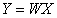(4)

In the above model, W is the inverse matrix of hybrid matrix A. Formula (8) is the generation model for . Formula (9) is the solution model for t . In Formula (9), when the Gauss feature of Y is stronger, Y is closer to the independent signal source S. While W is closer to inverse matrix of A.

2.2.2. ICA Solution Method

There are various ways to solve model, in which the objective function and optimization algorithm is usually applied. The objective function usually consists of kurtosis, negative entropy, and mutual information. And the optimization algorithm usually includes the gradient descent algorithm and the fast fixed-point algorithm . According to the central limit theory, the distribution of independent random variables tends to Gauss distribution under certain conditions. The sum of independent random variables is more close to the Gauss distribution than the original random variables and the maximum of non Gauss can be separated out from the independent source signals . This paper uses a method based on negative entropy of fast fixed-point algorithm, which is called Fast ICA algorithm. This algorithm has some good advantages such as: robustness and faster convergence rate. The algorithm is follows [13, 14, 15]:

(1)The measured data(x) is applied center and albino operator and the pretreated results is marked Z, randomly selecting the unit initialization vector w.

(2) Iteration, w← E {Zg (wTz)}- E {Zg′(wTz)} w, function g was defined as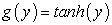(5)

(3) Normalization, w←w/| | w | |, which | | w | | is 2-norm of w;

(4) Back to the second step, it is iterated until that the 2-norm of difference of the adjacent is less than a given threshold value.

### 3. De-noising Method Based on the EMD-ICA

(1) Firstly, using the EMD method, the first derivative spectrum data x(t) is decomposed a series of IMFs.

(2) Then the successive order 3 IMF components are combined into a sequence sequentially, and the independent component analysis is applied for this sequence [15, 16]. For example: taking the first 3 order IMFs as a sequence then applying ICA analysis, the optimal analyzed results are seen as the first reconstruction component RIMF1. Nextly, the 2th, 3th, 4th order IMF are taken as a sequence and applied analysis to obtain the reconstructed component RIMF2, and so on. Finally, the n-2th, n-1th, nth order IMF are processed to get the RIMFn-2.

(3) After applying analysis, the n-2 reconstruction components (RIMF) can be obtained. Finally using the n-2 RIMFs to reconstruct the de-noised spectrum data, the available formula of de-noised spectrum data is written as :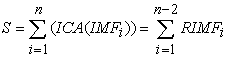(6)

S is the de-noised well logging signal. n is the total count of IMF. ICA (IMFi ) means applying ICA processing for the i th order IMF.

### 4. Evaluation Parameter of De-noising Effect

The good de-noising method should be that the de-noised spectrum curve remain shape and feature location, and that the curve is as smooth as possible. To investigate the de-noising effect for near infrared spectrum, five index of oil spectrum is used to evaluate the de-noising effect which consists of the signal to noise ratio (SNR), the mean square error (RMSE), smoothness index (SR), retention index of lateral characteristics (HFRI) and retention index of longitudinal characteristics (VFRI), Spectrum curve smoothing degree is measured by smoothness index SI. De-noising ability of algorithm is reflected by SNR, which its value is proportional to the de-noising effect. Differences of amplitude between the de-noised spectrum and the original spectrum are reflected by RMSE and its value is inversely to the de-noising effect. The feature preserving ability is measured by (HFRI) and (VFRI) at spectrum characteristic band. RMSE, SNR, SR, HFRI and VFRI is calculated [17, 18]:

Signal to noise ratio: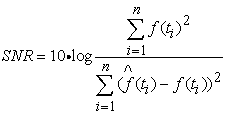(7)

Mean square error: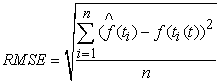(8)

Smoothness index: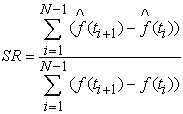(9)

Retention index of lateral characteristics:(10)

Retention index of longitudinal characteristics: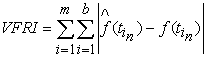(11)

### 5. Analysis of Experiments and Results

5.1. Spectrum Data

The Luminar 5030 with portable AOTF near infrared spectrometer, which is made in BRIMROSE company in the , is applied to collect spectrum data. Luminar 5030 is shown in Figure 1. Its wavelength range is from 1300 to 2300 nm, wavelength increment is 2 nm, scanning number of times is 600, and InGaAs detector is used. The near infrared spectrums of 48 fish oil samples are collected using diffuse reflectance spectroscopy measuring method and are shown in Figure 2(a). Figure 2(b) shows the first derivative spectrum of 48 fish oil samples. Filtering, PLSR and statistical analysis are realized in Unscrambler 9.8, Matlabnd Excel2003.

Figure 2. Original spectrum and first derivative spectrum of 48 fish oil samples (a) original spectrum (b) first derivative spectrum
5.2. De-noising of Fish Oil Spectrum

Figure 3 (a) shows the original spectrum of No.6 sample which can be seen that the information strength of the fish oil samples original spectra is low in full spectrum zone, and that the peak features of spectrum are not obvious, and that it is necessary to improve the spectrum resolution in order to promote the accuracy of spectrum analysis. The first derivative spectrum of No.6 sample is shown in Figure 3 (b). From the chart, it can be seen that derivative spectrum makes the spectrum peak become narrows, which makes spectrum characteristics (the zero-crossing of derivative spectrum and the extreme point of derivative spectra) easier to be seen. Therefore, derivative spectra are helpful in determining the position of peak accurately and can improve the spectrum analysis ability. But from the figure it can also be seen that the derivative spectrum also make the noise signal be enhanced, which reduces the SNR. In order to improve the accuracy of spectrum analysis, derivative spectrum must be de-noised.

Figure 3. Original spectrum and first derivative spectrum of No.6 sample (a) original spectrum (b) first derivative spectrum
Figure 4. Comparison of several de-noising methods for the spectrum of No.6 sample (a): Smoothing spectrum with window size 9 points; (b):Wavelet transform soft-threshold method; (c): EMD method (d): EMD+ICA method

Taking No.6 sample as example, De-noising analysis is executed for the near infrared spectroscopy of fish oil. The 9 point moving average smoothing is applied in spectrum smoothing method. Wavelet transform de-noising method used the "db5" wavelet with orthogonally and high order vanishing moments applying the 5-scale decomposition on the spectrum data. The wavelet soft threshold de-noising uses the Heursure threshold scheme for threshold estimation. EMD space-time filtering method uses soft threshold filtering method. Figure 4 is the de-noising effect comparison chart of derivative spectrum for No.6 sample, respectively processed by using the 9 point smoothing method, wavelet soft threshold method, EMD soft threshold filtering method and the EMD-ICA de-noising method.

For the quantitative evaluation of de-noising effect, the SNR, RMSE, SR, HFRI and VFRI are calculated. The three former parameters are calculated for all the bands, while HFRI and VFRI need to select the feature band position. According to the results shown in Figure 4, the apparent absorption band is seen as the location of the characteristic wavelengths. The selected characteristic absorption bands are 1396, 1718, 1765, 2109, 2230nm. Then the characteristic absorption band is taken as the center and around 5nm as the computed range. 5 bands are selected. Five evaluation parameters from several de-noising methods are listed in Table 1.

#### Table 1. Comparison of several de-noising methods for SNR, RMSE,SR, HFRI and VFRI

Some results can be drawn from Figure 4 and Table 1: (1) Spectrum smoothing method can effectively smooth high frequency noise and improve the SNR of spectrum, but also lead more effective information be lost. The retention ability of characteristic position, transverse or longitudinal, is poor. The smoothing effect and ability of maintaining characteristics are not good; (2) Compared with smoothing method, the wavelet threshold de-noising method and the EMD method are better. They have not much impact on the peak. And the SNR reaches more than 30dB after de-noising. While the RMSE is only about 0.003. The feature preservation is relatively better. In comparison, the EMD method has slightly better de-noising effect. (3) As to the de-noising effect, the proposed EMD-ICA method is better than other methods. Spectrum noise has been almost completely inhibited. The SNR reaches 34.613, while the root mean square error is only 0.00257. The smoothness index reaches 0.99976. EMD-ICA method also preserves characteristic peaks of spectrum data very good. It can be drawn that the EMD-ICA method has a better performance of detail preservation and de-noising.

To further highlight the de-noising effect of the proposed method, Figure 5 shows the de-noising results of the first derivative spectra of the 48 samples. Figure 5 (a) comes from the EMD method, Figure 5 (a) shows the results from EMD + ICA method proposed in this paper. Obviously, in Figure 5 (a) and (b), the spectrum peak is visualized, which is submerged by noise in Figure 2 (b). The signal-to-noise ratio is greatly enhanced. On the other hand, from the contrast of Figure 5 (a) and (b), it can be seen that the de-noising effect based on the EMD+ICA method is superior to that of the EMD method.

Figure 5. First derivative spectrum of 48 fish oil samples from different de-noising method, (a): EMD method (b): EMD+ICA method
5.3. DHA Content Detection

After 4 de-noising methods are applied, the related parameter prediction model is constructed using partial least squares regression (PLSR). By the procession of the model construction, full cross validation are sequenced (Leave-one-out) for all samples. The root means square error (RMSEP) and coefficient of determination (r2) of prediction is taken as a standard to evaluate the quality of the filtering method. There are 16 fish oil samples in the validation set. Their derivative spectrums are de-noised by using 3 kinds of filter method. Then the processed spectrums are taken as input variables of PLSR to detect DHA content.

Table 2 lists the comparison between the chemical value and the analysis results obtained from near infrared spectrum for the DHA content of fish oil. The near infrared spectrum comes from 3 pretreatment methods.

#### Table 2. Comparison of the prediction results of DHA content and chemical value by several de-noising methods

Compared with the 9-point smoothing, the decision coefficient (r2) of prediction set is increased from 0.9682 to 0.9887, the root mean square error (RMSEP) of prediction set is reduced from 0.0572 to0.0308. Compared with the wavelet and EMD methods, which is widely used in spectrum preprocessing, the treatment effect of EMD-ICA method is more ideal. The proposed method effectively improves the spectrum analysis precision.

### 6. Conclusions

This paper combined the empirical mode decomposition and independent component analysis into a new method and used it in the pretreatment of near infrared spectrum of fish oil. The principle and step of this method are given and the application effects of the method are evaluated. The experimental results show that the proposed method can retain details of fish oil spectrum data and attenuate noise greatly. After de-noising, the quantitative analysis model is established to determine the content of fish oil DHA. Detection results show that EMD-ICA method improved the detection accuracy of fish oil DHA content. It can be drawn that the proposed method effectively improves the quality of spectrum and future helps to establish model for fish oil component analysis and improve the model prediction precision.

### Acknowledgments

The authors wish to acknowledge the assistance and support of all those who contributed to our effort to enhance and develop the described system. The authors express their appreciation for the financial support provided by the National Natural Science Foundation of China (Project No: 41304098), Hunan Provincial Natural Science Foundation of China (Project No:12JJ4034), Young Scientific Research Fund of Hunan Provincial Education Department, PRC (Project No:13B076), Fund of the 11th Five-Year Plan for Key Construction Academic Subject (Optics) of Hunan Province, Optoelectronic information technology Hunan Province Talent training base between School and enterprise, and Hunan Province Key Laboratory of Photoelectric Information Integration and Optical Manufacturing Technology.

### Compliance with Ethics Requirements

Jianhua, Cai declares that he has no conflict of interest.

This article does not contain any studies with human or animal subjects.

### References

  Hans Buning-Pfaue. Analysis of water in food by near infrared spectroscopy. Food Chemistry, 2003, 82(1):107.In article CrossRef  Liu Jie, Li Xiaoyu, Li Peiwu,et al. Determination of moisture in chestnuts using near infrared spectroscopy. Transactions of the CSAE, 2010, 26(2):338.In article  Tan C,Li M L,Qin X. Random subspace regression ensemble for near-infrared spectroscopic calibration of tobacco samples. Analytical Sciences, 2008, 24(5):647.In article CrossRef  Jiang Rong, Yan Hong. Studies of spectral properties of short genes using the wavelet subspace Hilbert-Huang transform (WSHHT). Physica A, 2008, 387: 4223.In article CrossRef  Hao Yong, Chen Bin, Zhu Rui. Analysis of Several Methods for Wavelet De-noising Used in Near Infrared Spectrum Pretreatment, Spectroscopy and Spectral Analysis, 2006, 26(10):1838.In article  Cai Jianhua, Wang Xianchun. Near infrared spectrum pretreatment based on empirical mode decomposition. Acta Optica Sinica, 2010, 3 (1): 267.In article CrossRef  Huang N.E, Shen Z, Long S.R, et al. The empirical mode decomposition and the Hilbert spectrum for nonlinear and non-stationary time series analysis. Proc R Soc Lond Ser A, 1998, 454: 903.In article  Huang N E, Wu M L, Long S R, et al. A confidence limit for the empirical mode decomposition and Hilbert spectral analysis. Proceeding of Royal Society London A, 2003, 459: 2317.In article CrossRef  Cai,J.H, Tang,J,T, Hua,X,R. An analysis method for magnetotelluric data based on the Hilbert–Huang Transform, Exploration Geophysics, 2009, 40(2):197.In article CrossRef  BradleyM B, Camelia K. Application of the empirical mode decomposition and Hilbert-Huang transform to reflection seis-micdata. Geophysics, 2007, 72 (3): H29.In article  Hyvarinen A. Fast and robust fixed-point algorithms for independent component analysis. IEEE Transactions on Neural Networks, 1999,10(3):626.In article CrossRef  Peng Xuan,Yang Hongwei, Liu Jinfu, et al. A schur-lattice based linear ICA estimation algorithm. ACTA Electronic Sinica, 2004, 32(3):525.In article  Potamitis L, Fakotakis N, Kokkinakis G. Independent component analysis applied to feature extraction for robustautomatic speech recognition. Electronics letters, 9th November, 2000, 36(23): 1977.In article CrossRef  Asano F, Ikeda S, Ogawa M, et a.l Combined Approach of Array Processing and Independent Component Analysis for Blind Separation of Acoustic Signals. IEEE Transactions on Speech and Audio Processing. 2003, 11 (3): 204.In article CrossRef  Mijovic B, Dc Vos M, Uligorijcvic I, et al. Source separation from single-channel recordings by combining empirical mode decomposition and independent component analysis, IEEE transaction on Biomedical Engineering. 2010, 57(9):2188In article CrossRef  SUN Yun-lian, LUO Wei-hua, LI Hong. Extract Signals of Power Line Communication by a Novel Method Based on EMD and ICA, Proceedings of the CSEE, 2007, 27(16):109.In article  Wu Z, Huang N E. Ensemble empirical decomposition: a noised-assisted data analysis method, Advances in adaptive Data Analysis, 2009, 1(1):1In article  Chang K M. Ensemble empirical mode decomposition for high frequency ECG noise reduction. Biomedizinische Technik, 2010, 55(4):193.In article CrossRef